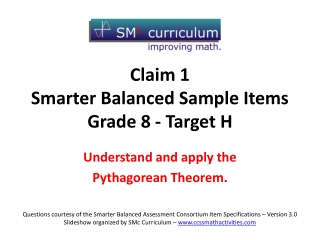DownloadDownload PresentationClaim 1 Smarter Balanced Sample Items Grade 8 - Target H

# Claim 1 Smarter Balanced Sample Items Grade 8 - Target H

Télécharger la présentation## Claim 1 Smarter Balanced Sample Items Grade 8 - Target H

- - - - - - - - - - - - - - - - - - - - - - - - - - - E N D - - - - - - - - - - - - - - - - - - - - - - - - - - -
##### Presentation Transcript

1. Claim 1Smarter Balanced Sample ItemsGrade 8 - Target H Understand and apply the Pythagorean Theorem. Questions courtesy of the Smarter Balanced Assessment Consortium Item Specifications – Version 3.0 Slideshow organized by SMc Curriculum – www.ccssmathactivities.com

2. #1 A right triangle is shown. Enter the value of x.

3. #1 Answer Rubric: (1 point) Student enters correct value. Answer: 2.6

4. #2 A right square pyramid is shown. The height of the pyramid is 4 units. The distance from the center of the base of the pyramid to vertex D is 3 units, as shown. Enter the length of segment AD, in units.

5. #2 Answer Rubric: (1 point) Student enters correct value. Answer: 5

6. #3 A 13-foot ladder is leaning on a tree. The bottom of the ladder is on the ground at a distance of 5 feet from the base of the tree. The base of the tree and the ground form a right angle as shown. Enter the distance between the ground and the top of the ladder, x, in feet.

7. #3 Answer Rubric: (1 point) Student enters correct value. Answer: 12

8. #4 The table shows the side lengths for some triangles. Determine whether the side lengths define a right triangle. Select Yes if it is a right triangle. Select No if it cannot be a right triangle.

9. #4 Answer Rubric: (1 point) Student correctly classifies triangles. Answer: N, N, Y

10. #5 A coordinate plane is shown with labeled points. What is the distance between point A and point B on the coordinate plane? A. 5 B. 6 C. 10 D. 14

11. #5 Answer Rubric: (1 point) Student selects the distance between point A and point B. Answer: C

12. #6 • What is the distance between points • (5, 2) and (-3, -4) on the coordinate plane? • 5 • 6 • 10 • 14

13. #6 Answer Rubric: (1 point) Student selects the distance between point A and point B. Answer: C

14. #7 The points show different locations in Joe’s town. Each unit represents 1 mile. What is the distance, in miles, between Joe’s Home and the Park? Round your answer to the nearest tenth.

15. #7 Answer Rubric: (1 point) The student finds the distance. Answer: 3.6